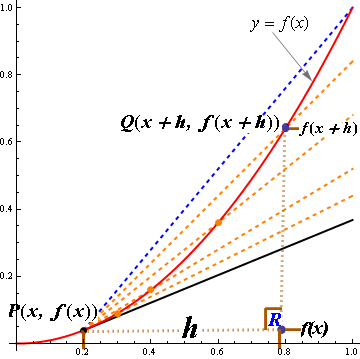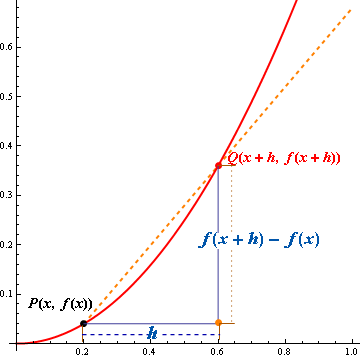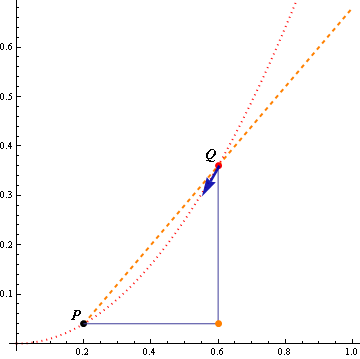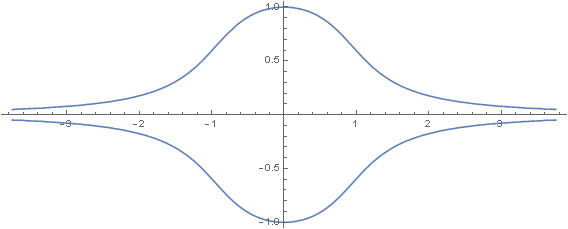$$\diamondsuit$$Please double click any math symbols to enlarge then a single click will dismiss.

We have learned to take derivative of a function at a point. Now we need to distinguish the following:

Derivative at a point $$x=a$$

$$f'(a)=\lim\limits_{h\to 0}\frac{f(a+h)-f(a)}{h}$$

Derivative as a function (arbitrary points)

$$f'(x)=\lim\limits_{h\to 0}\frac{f(x+h)-f(x)}{h}$$The following may help you to memorize the first principle and the concept of derivative:

• Slope of secant line approaches to slope of the tangent line (see the picture)
• Limit of the ratio of change: $$\frac{\text{Change of } y}{\text{Change of }x } \text{ or use notation } \ \frac{\Delta y}{\Delta x}$$ to help you memorize.

Notations for derivative (for a function $$y=f(x)$$):

For the derivative function, we can use any one of the following notations

$$y', \ f'(x),\ \frac{dy}{dx},\ \frac{df}{dx},\ \frac{df(x)}{dx},\ \frac{d}{dx} f(x).$$

For derivative at a particular point $$x=a$$, we can use any one of the following notations

$$y'(a),\ y'|_{x=a}, \ f'(a),\ \left.\frac{dy}{dx}\right|_{x=a},\ \left.\frac{df}{dx}\right|_{x=a},\ \left.\frac{df(x)}{dx}\right|_{x=a},\ \left.\frac{d}{dx} f(x)\right|_{x=a}.$$

A further comment:

Around 1950, a MIT Professor, Struik Dirk, taught his student in this way about tangent line:"The tangent passes through two consecutive points of the curve".

As for computer graphics, they consists of pixels, which can be think of as some tiny dots. A secant line passing two adjacent pixels on a curve would be a very good approximation of a tangent line. The following picture will illustrate  the idea, if you can imagine that Q approaches to P all the way to the closest dot on the curve (dotted approximation of the continuous curve) .

As an exercise, use the function $$y=x^2$$, take a sequence of values in the following as the $$x$$ coordinates of the move point Q:

1, 0.8, 0.6, 0.4, 0.3, 0.25, 0.21, 0.201,

then calculate the corresponding $$y$$ values and equation of the corresponding secant lines and their slopes. After that, construct a table and observe that the slope of the secant lines indeed approaches to the slope of the tangent line and the derivative $$f'(0.2)$$.

______________________________________________________________________________________

Example (Implicit differentiation):

$$\underline{x^4 y^2}+y^2 \left(x^2+y^2\right)^2=1$$. Find the slope of tangent at $$P(0,1)$$

Solution:  $$\underline{4x^3y^2+x^4(2y\cdot\frac{dy}{dx})}+2y\cdot\frac{dy}{dx}\cdot \left(x^2+y^2\right)^2+ y^2\cdot 2\left(x^2+y^2\right)\left(2x+2y\frac{dy}{dx}\right)=0$$

$$2x^3y^2+x^4y\cdot\frac{dy}{dx}+y\left(x^2+y^2\right)^2\cdot\frac{dy}{dx}+ 2\left(x^2+y^2\right)y^2\left(x+y\frac{dy}{dx}\right)=0$$.

Plug in $$x=0, y=1$$ above to get $$\left.\frac{dy}{dx}\right|_{P(0,1)}=0$$.

In general, $$2x^3y^2+x^4y\cdot\frac{dy}{dx}+y\left(x^2+y^2\right)^2\cdot\frac{dy}{dx}+ 2xy^2 \left(x^2+y^2\right)+2y^3\left(x^2+y^2\right)\cdot \frac{dy}{dx}=0$$.

Solve for $$\frac{dy}{dx}$$, get $$\frac{dy}{dx}=-\frac{2xy^2(2x^2+y^2)}{x^4y+y(x^2+y^2)^2+2y^3(x^2+y^2)}$$

The picture of the curve looks like this:.